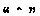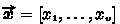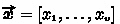# Polynomial Representation Is Tricky: Maliciously Secure Private Set Intersection Revisited

• 1229 Accesses

Part of the Lecture Notes in Computer Science book series (LNSC,volume 12973)

## Abstract

Private Set Intersection protocols (PSIs) allow parties to compute the intersection of their private sets, such that nothing about the sets’ elements beyond the intersection is revealed. PSIs have a variety of applications, primarily in efficiently supporting data sharing in a privacy-preserving manner. At Eurocrypt 2019, Ghosh and Nilges proposed three efficient PSIs based on the polynomial representation of sets and proved their security against active adversaries. In this work, we show that these three PSIs are susceptible to several serious attacks. The attacks let an adversary (1) learn the correct intersection while making its victim believe that the intersection is empty, (2) learn a certain element of its victim’s set beyond the intersection, and (3) delete multiple elements of its victim’s input set. We explain why the proofs did not identify these attacks and propose a set of mitigations.

This is a preview of subscription content, access via your institution.

Chapter
USD   29.95
Price excludes VAT (USA)
• DOI: 10.1007/978-3-030-88428-4_35
• Chapter length: 22 pages
• Readable on all devices
• Own it forever
• Exclusive offer for individuals only
• Tax calculation will be finalised during checkout
eBook
USD   89.00
Price excludes VAT (USA)
• ISBN: 978-3-030-88428-4
• Readable on all devices
• Own it forever
• Exclusive offer for individuals only
• Tax calculation will be finalised during checkout
Softcover Book
USD   119.99
Price excludes VAT (USA)
1. 1.

To lower the polynomial multiplication cost to $$O(m\log _{\scriptscriptstyle 2}m)$$, one may use Fast Fourier Transform (FFT). However, as FFT uses point-value polynomial representation, further security analysis is required to ensure the attack would not be enabled again.

## References

1. Abadi, A., Terzis, S., Metere, R., Dong, C.: Efficient delegated private set intersection on outsourced private datasets. IEEE TDSC (2018)

2. Abadi, A., Murdoch, S.J., Zacharias, T.: Polynomial representation is tricky: Maliciously secure private set intersection revisited (Full version) (2021). https://eprint.iacr.org/2021/1009.pdf

3. Abadi, A., Terzis, S., Dong, C.: O-PSI: delegated private set intersection on outsourced datasets. In: IFIP SEC (2015)

4. Abadi, A., Terzis, S., Dong, C.: VD-PSI: verifiable delegated private set intersection on outsourced private datasets. In: FC (2016)

5. Abadi, A., Terzis, S., Dong, C.: Feather: Lightweight multi-party updatable delegated private set intersection. IACR Cryptology ePrint Archive (2020)

6. Aho, A.V., Hopcroft, J.E.: The Design and Analysis of Computer Algorithms. Addison-Wesley Longman Publishing Co., Inc., Boston (1974)

7. Ben-Efraim, A., Nissenbaum, O., Omri, E., Paskin-Cherniavsky, A.: Psimple: Practical multiparty maliciously-secure private set intersection, ePrint Archive (2021)

8. Boneh, D., Gentry, C., Halevi, S., Wang, F., Wu, D.J.: Private database queries using somewhat homomorphic encryption. In: ACNS (2013)

9. Brickell, J., Porter, D.E., Shmatikov, V., Witchel, E.: Privacy-preserving remote diagnostics. In: CCS (2007)

10. Bursztein, E., Hamburg, M., Lagarenne, J., Boneh, D.: Openconflict: preventing real time map hacks in online games. In: IEEE S&P (2011)

11. Camenisch, J., Zaverucha, G.M.: Private intersection of certified sets. In: FC (2009)

12. Canetti, R.: Universally composable security: a new paradigm for cryptographic protocols. In: FOCS (2001)

13. Chase, M., Miao, P.: Private set intersection in the internet setting from lightweight oblivious PRF. In: CRYPTO (2020)

14. Chen, H., Laine, K., Rindal, P.: Fast private set intersection from homomorphic encryption. In: CCS (2017)

15. Cristofaro, E.D., Lu, Y., Tsudik, G.: Efficient techniques for privacy-preserving sharing of sensitive information. In: TRUST (2011)

16. Duong, T., Phan, D.H., Trieu, N.: Catalic: delegated PSI cardinality with applications to contact tracing. In: ASIACRYPT (2020)

17. Freedman, M.J., Nissim, K., Pinkas, B.: Efficient private matching and set intersection. In: EUROCRYPT (2004)

18. Ghosh, S., Nielsen, J.B., Nilges, T.: Maliciously secure oblivious linear function evaluation with constant overhead. In: ASIACRYPT (2007)

19. Ghosh, S., Nilges, T.: An algebraic approach to maliciously secure private set intersection (full version). ePrint Archive (2017). https://eprint.iacr.org/2017/1064

20. Ghosh, S., Nilges, T.: An algebraic approach to maliciously secure private set intersection. In: EUROCRYPT (2019)

21. Ghosh, S., Simkin, M.: The communication complexity of threshold private set intersection. In: CRYPTO (2019)

22. Hazay, C., Venkitasubramaniam, M.: Scalable multi-party private set-intersection. In: PKC (2017)

23. Inbar, R., Omri, E., Pinkas, B.: Efficient scalable multiparty private set-intersection via garbled bloom filters. In: SCN (2018)

24. Ion, M., et al.: On deploying secure computing: private intersection-sum-with-cardinality. In: IEEE EuroS&P (2020)

25. Ishai, Y., Kushilevitz, E.: Randomizing polynomials: a new representation with applications to round-efficient secure computation. In: FOCS (2000)

26. Kamara, S., Mohassel, P., Raykova, M., Sadeghian, S.: Scaling private set intersection to billion-element sets. In: FC (2014)

27. Katz, J., Myers, S.A., Ostrovsky, R.: Cryptographic counters and applications to electronic voting. In: EUROCRYPT (2001)

28. Kissner, L., Song, D.X.: Privacy-preserving set operations. In: CRYPTO (2005)

29. Kolesnikov, V., Kumaresan, R., Rosulek, M., Trieu, N.: Efficient batched oblivious PRF with applications to private set intersection. In: CCS (2016)

30. Kolesnikov, V., Matania, N., Pinkas, B., Rosulek, M., Trieu, N.: Practical multi-party private set intersection from symmetric-key techniques. In: CCS (2017)

31. Pinkas, B., Rosulek, M., Trieu, N., Yanai, A.: PSI from PaXoS: fast, malicious private set intersection. In: EUROCRYPT (2020)

32. Quarteroni, A., Sacco, R., Saleri, F.: Numerical Mathematics, vol. 37. Springer Science & Business Media, Heidelberg (2010)

33. Reed, I.S., Solomon, G.: Polynomial codes over certain finite fields. J. Soc. Ind. Appl. Math. 8(2), 300–304 (1960)

34. Shamir, A.: How to share a secret. Commun. ACM 22(11), 612–613 (1979)

35. Thomas, K., et al.: Protecting accounts from credential stuffing with password breach alerting. In: USENIX Security (2019)

36. Yang, X., Luo, X., Wang, X.A., Zhang, S.: Improved outsourced private set intersectionprotocol based on polynomial interpolation. Concurr. Comput. Pract. Exp. (2018)

37. Zhang, E., Liu, F., Lai, Q., Jin, G., Li, Y.: Efficient multi-party private set intersection against malicious adversaries. In: CCSW (2019)

38. Zhao, Y., Chow, S.S.M.: Can you find the one for me? Privacy-preserving matchmaking via threshold PSI. ePrint Archive (2018)

## Acknowledgments

Aydin Abadi is supported by REPHRAIN: The National Research Centre on Privacy, Harm Reduction and Adversarial Influence Online, under UKRI grant: EP/V011189/1. Steven J. Murdoch is supported by The Royal Society under grant UF160505. Thomas Zacharias is supported by the Horizon 2020 project # 780477 (PRIViLEDGE).

## Author information

Authors

### Corresponding author

Correspondence to Aydin Abadi .

## Appendices

### A Identified Flaws In The Security Proofs

Below, we briefly explain a set of flaws we identified in the security proofs of the paper’s conference  and full  versions. These flaws made the three attacks undetected. We categorise the flaws in three classes based on their relevance to each attack. For the sake of simplicity, we exclude the hat symbol,, used in the original proofs. See our paper’s full version  for a more detailed analysis.

### 1.1 A.1 Class 1: Not All Checks Have Been Included

In this section, we describe a flaw in the proof of two-party PSI (page 20 in ) that lets the environment use Attack 1 to distinguish the two worlds. Briefly, the flaw is that the proof does not consider the case where $$\delta ^{\scriptscriptstyle *}_{\scriptscriptstyle B}\ne \mathbf {r}'_{\scriptscriptstyle B}(z)$$. Before we elaborate on it, we highlight two typos in “Hybrid” 1; namely, $${\alpha }^{\scriptscriptstyle *}_{\scriptscriptstyle A} \ne \mathbf {p}_A(z)$$ and $${\beta }^{\scriptscriptstyle *}_{\scriptscriptstyle A} \ne \mathbf {r}_{\scriptscriptstyle A}(z)$$ should have been $${\alpha }^{\scriptscriptstyle *}_{\scriptscriptstyle B} \ne \mathbf {p}_{\scriptscriptstyle B}(z)$$ and $${\beta }^{\scriptscriptstyle *}_{\scriptscriptstyle B} \ne \mathbf {r}_{\scriptscriptstyle B}(z)$$ respectively, as the proof is for corrupt party B. In Hybrid 2, it is stated that “an environment distinguishing Hybrid 1 and 2 must manage to send $$\mathbf {p}^{\scriptscriptstyle *}_{\scriptscriptstyle \cap }$$ such that $$\mathbf {p}^{\scriptscriptstyle *}_{\scriptscriptstyle \cap } \ne \mathbf {p}_{\scriptscriptstyle A}\cdot (\mathbf {r}_{\scriptscriptstyle B}+\mathbf {r}'_{\scriptscriptstyle A}) + \mathbf {p}_{\scriptscriptstyle B}\cdot (\mathbf {r}'_{\scriptscriptstyle B}+\mathbf {r}_{\scriptscriptstyle A})$$ while passing the check in Step 5 [of Fig. 9] with non-negligible probability.” The proof shows that the check fails only in the cases where $${\alpha }^{\scriptscriptstyle *}_{\scriptscriptstyle B} \ne \mathbf {p}_{\scriptscriptstyle B}(z)$$ and $${\beta }^{\scriptscriptstyle *}_{\scriptscriptstyle B} \ne \mathbf {r}_{\scriptscriptstyle B}(z)$$; therefore, $$\delta ^{\scriptscriptstyle *}_{\scriptscriptstyle B}\ne \mathbf {r}'_{\scriptscriptstyle B}(z)$$ has been left out of the proof. The lack of such analysis leads to the following issue. As we have shown, the check does not fail for certain $$\mathbf {p}_{\scriptscriptstyle \cap }$$ and $$\delta _{\scriptscriptstyle B}$$ such that $$\mathbf {p}_{\scriptscriptstyle \cap } \ne \mathbf {p}_{\scriptscriptstyle A}\cdot (\mathbf {r}_{\scriptscriptstyle B}+\mathbf {r}'_{\scriptscriptstyle A}) + \mathbf {p}_{\scriptscriptstyle B}\cdot (\mathbf {r}'_{\scriptscriptstyle B}+\mathbf {r}_{\scriptscriptstyle A})$$ and $$\delta _{\scriptscriptstyle B}\ne \mathbf {r}'_{\scriptscriptstyle B}(z)$$. So, the adversary can pass the check with a high probability in the real world (or Hybrid 0). The simulator, in Hybrid 2, detects this inconsistency (i.e., $$\delta ^{\scriptscriptstyle *}_{\scriptscriptstyle B}\ne \mathbf {r}'_{\scriptscriptstyle B}(z)$$) according to Fig. 11 in . But, the simulator in Hybrid 1 cannot detect it, as it only aborts if $${\alpha }^{\scriptscriptstyle *}_{\scriptscriptstyle B} \ne \mathbf {p}_{\scriptscriptstyle B}(z)$$ or $${\beta }^{\scriptscriptstyle *}_{\scriptscriptstyle B} \ne \mathbf {r}_{\scriptscriptstyle B}(z)$$. Thus, Hybrids 1 and 2 (likewise Hybrids 0 and 2) are distinguishable by the environment.

### 1.2 A.2 Class 2: Incomplete Simulator

In the proof of Lemma 4.1, i.e., OPA’s security, in the paper’s conference version , it is stated that “the only possibility for an environment to distinguish between the simulation and the real protocol is by succeeding in answering the check while using a malformed input, i.e. a polynomial of incorrect degree or 0-polynomials.” We argue that this is not the only possible case. As we indicated in Attack 2, it is possible the adversary (in the real world) in the “consistency check” phase, deviates from the protocol and still passes the verification. This will ultimately let the environment distinguish the two worlds. Note, the proof should have included the simulation of the “consistency check” phase. Accordingly, the proof does not capture the case where $$w'$$ of the form $$w'\ne \mathbf {r}(x'^{\scriptscriptstyle *})$$ is used by the adversary. In the simulation of the consistency check, the simulator can detect when it is given $$w'\ne \mathbf {r}(x'^{\scriptscriptstyle *})$$, as it has already extracted polynomial $$\mathbf {r}$$ from the adversary. But, in the real world, as we have shown, the adversary can pass the check when $$w'\ne \mathbf {r}(x'^{\scriptscriptstyle *})$$ and a certain value, $$x'^{\scriptscriptstyle *}$$, is used in this phase. Hence, the environment can distinguish the two worlds. This issue arises because the proof does not analyse the case where the check, in the consistency check phase, is passed but $$w'\ne \mathbf {r}(x'^{\scriptscriptstyle *})$$ is used in this phase.

### 1.3 A.3 Class 3: Incomplete Definition Of Malformed Input

Recall, the proof of Lemma 4.1 considers a malformed input if an input polynomial is (i) of incorrect degree or (ii) zero. The issue is that the proof shows only in these two cases the environment cannot distinguish the two worlds. We argue that an input can be malformed without satisfying conditions (i) or (ii). Similar to the description of Attack 3, let a corrupt sender (for all $$j\in [2d+1]$$) send $$q_{\scriptscriptstyle 3,j}=\bar{\mathbf {r}}_{\scriptscriptstyle A}(x_{\scriptscriptstyle j})\cdot (x_{\scriptscriptstyle j}-s^{\scriptscriptstyle (B)}_{\scriptscriptstyle 1})^{\scriptscriptstyle -1}$$ to $$\mathcal {F}^{\scriptscriptstyle (j)}_{\scriptscriptstyle \text {OLE}^{\scriptscriptstyle +}}$$ in the ideal world. This lets the simulator obtain all $$q_{\scriptscriptstyle 3,j}$$ and interpolate a polynomial, $$\mathbf {q}$$. There would be two cases: (1) $$deg(\mathbf {q})>d$$, or (2) $$deg(\mathbf {q})\le d$$. In case (1), the simulator aborts. But in the real protocol (in step 1b of Fig. 1) the honest party never aborts. Because, in general, polynomial $$\mathbf {s}$$ interpolated from $$2d+1$$ pairs $$(x_{\scriptscriptstyle j},s_{\scriptscriptstyle j})$$ always has degree at most 2d by Theorem 2. This issue lets the environment distinguish the two worlds. Now we move on to case (2). In the ideal world, in the consistency check phase, the simulator of the OPA is given random value $$x^{\scriptscriptstyle *}$$ and $$w''=\bar{\mathbf {r}}_{\scriptscriptstyle A}(x^{\scriptscriptstyle *})\cdot (x^{\scriptscriptstyle *}-s^{\scriptscriptstyle (B)}_{\scriptscriptstyle 1})^{\scriptscriptstyle -1}$$ and wants to check $$w''{\mathop {=}\limits ^{\scriptscriptstyle ?}}\mathbf {q}(x^{\scriptscriptstyle *})$$. Note, the equation may not always hold; because factors $$(x_{\scriptscriptstyle j}-s^{\scriptscriptstyle (B)}_{\scriptscriptstyle 1})^{\scriptscriptstyle -1}$$ of y-coordinates $$q_{\scriptscriptstyle 3,j}$$ from which $$\mathbf {q}$$ was interpolated, are not directly generated by evaluating a polynomial at $$x_{\scriptscriptstyle j}$$’s. The probability that $$w''=\mathbf {q}(x^{\scriptscriptstyle *})$$ depends on the choice of $$x^{\scriptscriptstyle *}$$. If the equation holds, then the simulator does not abort; also, the honest party does not abort as we showed in Attack 3. This is problematic, as the attack has been successfully mounted without being detected in both worlds. If $$w''\ne \mathbf {q}(x^{\scriptscriptstyle *})$$, the simulator aborts, but the honest party does not abort, as the adversary can pass the consistency check. So, the environment can distinguish the two worlds. This issue arises because, in the proof, the definition of a malformed input has been limited to only the above conditions (i) and (ii), and the proof never analyses the case where the check is passed while $$w''$$ (s.t., $$w''\ne \mathbf {r}(x'^{\scriptscriptstyle *}))$$ is sent to $$\mathcal {F}_{\scriptscriptstyle \text {OLE}}^{\scriptscriptstyle 2}$$.

The adversary in Attack 3, can pass the PSI’s verification too. The issue is that in the PSI’s proof (i.e., proof of Theorem 5.1 in ) when A is corrupt, the case where $$\beta _{\scriptscriptstyle A}$$ is not the result of evaluating truly random polynomial $$\mathbf {r}_{\scriptscriptstyle A}$$ at z (i.e., $$\beta _{\scriptscriptstyle A}\ne \mathbf {r}_{\scriptscriptstyle A}(z)$$) is never analysed in detail and also it is assumed that the only way the adversary changes the original value is via a modular addition (i.e., $$\alpha _{\scriptscriptstyle A}+e$$); so, a modular multiplication is never considered as a part of the attack. But, as we showed, the adversary can multiply its input y-coordinates by certain values to affect the result’s correctness and pass the verification.

### B Attack 3 Theorems

We first restate Theorem 2 that will be used by the main one, i.e., Theorem 1.

### Theorem 2

(Uniqueness of interpolating polynomial ). Letbe a vector of non-zero distinct elements. For v arbitrary values: $$y_{\scriptscriptstyle 1},\ldots ,y_{\scriptscriptstyle v}$$ there is a unique polynomial: $$\boldsymbol{\tau }$$, of degree at most $$v-1$$ such that: $$\forall j, 1\le j\le v: \boldsymbol{\tau }(x_{\scriptscriptstyle j})=y_{\scriptscriptstyle j}$$, where $$x_{\scriptscriptstyle j},y_{\scriptscriptstyle j}\in \mathbb {F}$$.

Informally, Theorem 1 states that a set of y-coordinates of a polynomial can be multiplied by a set of non-zero values, such that the polynomial interpolated from the product misses a specific root of the original polynomial.

### Theorem 1

Letbe a vector of non-zero distinct elements. Let $$\boldsymbol{\mu }=\prod \limits ^{\scriptscriptstyle \ddot{o}}_{\scriptscriptstyle i=1}(x-e_{\scriptscriptstyle i})\in \mathbb {F}[X]$$ be a degree $$\ddot{o}< v$$ polynomial with $$\ddot{o}$$ distinct roots $$e_{\scriptscriptstyle 1},\ldots ,e_{\scriptscriptstyle \ddot{o}}$$, and let $$\mu _{\scriptscriptstyle j}=\boldsymbol{\mu }(x_{\scriptscriptstyle j}),$$ where $$1\le j \le v$$. For some $$c\in [\ddot{o}]$$ such that $$e_{\scriptscriptstyle c}\notin \{x_{\scriptscriptstyle 1},\ldots ,x_{\scriptscriptstyle v}\}$$, let $$\boldsymbol{\mu }'$$ be a degree $$\ddot{o}-1$$ polynomial interpolated from pairs $$(x_{\scriptscriptstyle 1}, \mu _{\scriptscriptstyle 1}\cdot (x_{\scriptscriptstyle 1}-e_{\scriptscriptstyle c})^{\scriptscriptstyle -1} ),..., (x_{\scriptscriptstyle v}, \mu _{\scriptscriptstyle v}\cdot (x_{\scriptscriptstyle v}-e_{\scriptscriptstyle c})^{\scriptscriptstyle -1} )$$. Then, $$\boldsymbol{\mu }'$$ will not have $$e_{\scriptscriptstyle c}$$ as root, i.e. $$\boldsymbol{\mu }'(e_{\scriptscriptstyle c})\ne 0$$.

### Proof

For the sake of simplicity and without loss of generality, let $$c=1$$. We can rewrite polynomial $$\boldsymbol{\mu }$$ as $$\boldsymbol{\mu }(x)=(x-e_{\scriptscriptstyle 1})\cdot \prod \limits ^{\scriptscriptstyle \ddot{o}}_{\scriptscriptstyle i=2}(x-e_{\scriptscriptstyle i})$$. Then, every $$\mu _{\scriptscriptstyle j}$$ ($$1\le j \le v$$) can be written as: $$\mu _{\scriptscriptstyle j}=(x_{\scriptscriptstyle j}-e_{\scriptscriptstyle 1})\cdot \prod \limits ^{\scriptscriptstyle \ddot{o}}_{\scriptscriptstyle i=2}(x_{\scriptscriptstyle j}-e_{\scriptscriptstyle i})$$. Accordingly, for every j, the product $$\alpha _{\scriptscriptstyle j}:=\mu _{\scriptscriptstyle j}\cdot (x_{\scriptscriptstyle j}-e_{\scriptscriptstyle 1})^{\scriptscriptstyle -1}$$ has the form: $$\alpha _{\scriptscriptstyle j}=\mu _{\scriptscriptstyle j}\cdot (x_{\scriptscriptstyle j}-e_{\scriptscriptstyle 1})^{\scriptscriptstyle -1}=\prod \limits ^{\scriptscriptstyle \ddot{o}}_{\scriptscriptstyle i=2}(x_{\scriptscriptstyle j}-e_{\scriptscriptstyle i})$$. Let $$\boldsymbol{\mu }''$$ be a degree $$\ddot{o}-1$$ polynomial with $$\ddot{o}-1$$ distinct roots identical to the roots of $$\boldsymbol{\mu }$$ excluding $$e_{\scriptscriptstyle 1}$$, i.e., $$\boldsymbol{\mu }''(e_{\scriptscriptstyle 1})\ne 0$$. By the Polynomial Remainder Theorem, $$\boldsymbol{\mu }''$$ can be written as $$\boldsymbol{\mu }''(x)=K\cdot \prod \limits ^{\scriptscriptstyle \ddot{o}}_{\scriptscriptstyle i=2}(x-e_{\scriptscriptstyle i})$$, where $$K\in \mathbb {F}\setminus \{0\}$$. So, it holds that $${\forall }j\in [v]: \mu ''(x_{\scriptscriptstyle j})=K\cdot \prod \limits ^{\scriptscriptstyle \ddot{o}}_{\scriptscriptstyle i=2}(x_{\scriptscriptstyle j}-e_{\scriptscriptstyle i})=K\cdot \alpha _{\scriptscriptstyle j}.$$ This implies that $$\boldsymbol{\mu }''$$ is a degree $$\ddot{o}-1$$ polynomial interpolated from $$(x_{\scriptscriptstyle 1},K\cdot \alpha _{\scriptscriptstyle 1}),\ldots ,(x_{\scriptscriptstyle v},K\cdot \alpha _{\scriptscriptstyle v})$$. By its definition, the polynomial $$\boldsymbol{\mu }'$$ is interpolated from the pairs $$(x_{\scriptscriptstyle 1},\alpha _{\scriptscriptstyle 1}),\ldots ,(x_{\scriptscriptstyle v},\alpha _{\scriptscriptstyle v})$$. Thus, $$K\cdot \boldsymbol{\mu }'$$ is another degree $$\ddot{o}-1$$ polynomial interpolated from $$(x_{\scriptscriptstyle 1},K\cdot \alpha _{\scriptscriptstyle 1}),\ldots ,(x_{\scriptscriptstyle v},K\cdot \alpha _{\scriptscriptstyle v})$$. Due to Theorem 2, we have that $$\boldsymbol{\mu }''=K\cdot \boldsymbol{\mu }'$$, so $$\boldsymbol{\mu }''(e_{\scriptscriptstyle 1})=K\cdot \boldsymbol{\mu }'(e_{\scriptscriptstyle 1})\Rightarrow \boldsymbol{\mu }'(e_{\scriptscriptstyle 1})=K^{\scriptscriptstyle -1}\cdot \boldsymbol{\mu }''(e_{\scriptscriptstyle 1})$$. We also know that $$K^{\scriptscriptstyle -1}\ne 0$$ and $$\boldsymbol{\mu }''(e_{\scriptscriptstyle 1})\ne 0$$. Since $$\mathbb {F}$$ is an integral domain, it follows that $$\boldsymbol{\mu }'(e_{\scriptscriptstyle 1})=K^{\scriptscriptstyle -1}\cdot \boldsymbol{\mu }''(e_{\scriptscriptstyle 1})\ne 0$$.

$$\square$$

## Rights and permissions

Reprints and Permissions